Get our FREE VBA eBook of the 30 most useful Excel VBA macros.

Automate Excel so that you can save time and stop doing the jobs a trained monkey could do.

# Convert color codes (RGB, HSL, Hex, Long, CMYK)I often find myself converting between different formats of color codes; especially from hex to RGB and back again.  Rather than firing up Google and searching for color conversion tools online, I decided to write my own User Defined Functions in Excel, now I can calculate the result within a worksheet instead.

The functions in this post include the conversions for RGB, hex, HSL, CMYK and long formats.

Using these color conversion functions, I created an add-in which makes the conversion even easier.  Keep reading to find out how you can download the add-in for free.

I recommend you download the example file for this post.  Then you’ll be able to work along with examples and see the solution in action, plus the file will be useful for future reference.

Contents

## Excel’s different color code formats

Even in Excel, there are four different methods of defining color; RGB, hex, HSL and long.

### RGB

The RGB (Red, Green and Blue) color code is used within the standard color dialog box.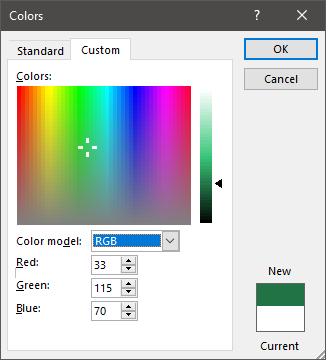The individual colors Red, Green and Blue each have 256 different shades, which when mixed can create 16,777,216 different color combinations.

### HSL

Within the standard color dialog box there is another code format; using the color model drop-down we can change to HSL.  HSL uses Hue, Saturation and Luminance to create the color.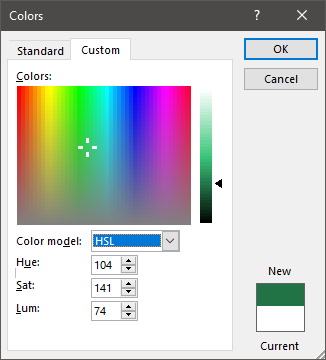HSL tries to define colors closer to the way humans think about colors.

• Hue – is the degree on the color wheel from 0 to 360.  0 is red, 120 is green, 240 is blue.
• Saturation – is a percentage of the amount of color included.  100% is full color, 0% is no color (i.e. all grey)
• Luminance (or lightness) – is a percentage of grey.  0% is black, 100% is white.

Frustratingly, Excel does not handle HSL in the standard way.  Instead, Excel measures all the numbers where 0 is the lowest and 255 is the biggest.  But, it’s a quirk we can handle.

### Long

The long color code is used by VBA when displaying the color property of an item.  The following macro displays the long code for the fill of the active cell.

```Sub ActiveCellColor()

MsgBox "Long color code: " & ActiveCell.Interior.Color

End Sub```

Select a cell and run the macro.The long code is a number from 0 to 16,777,215, where each separate number represents a color.  The relationship between RGB and long is based on a simple calculation:

Long = Blue x 256 x 256 + Green x 256 + Red

As an example:

• Where Red: 33, Green: 115 and Blue: 70
• The result calculates as 70 x 256 x 256 + 115 x 256 + 33    =    4,616,993

Long and RGB are related; they are just different ways of calculating the same number.

### Hex

Hex color codes are similar to RGB as they also use 256 shades for each individual color.  The critical difference is that the hex system represents the numbers from 0 to 255 using just two characters.  This is possible because hex uses Base-16.

We generally use Base-10 in everyday life, which means that we have 10 digits (0, 1, 2, 3, 4, 5, 6, 7, 8, 9) available.  As an example, the number 14 is created by using the formula (1 x base) + 4.  The base is 10, so the result is calculated as  (1 x 10) + 4, which equals 14.

For Base-16, there are 16 digits; however, as we don’t have enough number characters we use A, B, C, D, E and F to represent the numbers from 10 through 15.

As an example, the hex number AB equals 171.

• A = 10
• B = 11.
• The calculation is (A x base) + B   =     (10 x 16) + 11   =    171

In hex, the lowest value is zero and the highest value is F (which is equal to  15).  Therefore, there are 16 possible individual digits.  This means that from two digits we can create 256 shades (16 x 16 = 256), which is the same as RGB.  Therefore, hex also has the same 16,777,216 combinations as RGB and long.

Hex codes are presented in same order to RGB, so if the hex color code is #467321 the first two characters represent red, the middle two characters represent green and the last two are blue.

Using the #467321 hex code as an example, the conversion to RGB would be:

• Red: Hex  value of 46 calculates as (4 * 16) + 6 = 70
• Green: Hex value of 73 calculates as (7 * 16) + 3 = 115
• Blue: Hex value of 21 calculates as (2 * 16) + 1 = 33

The hex codes are used within the VBA properties to define color.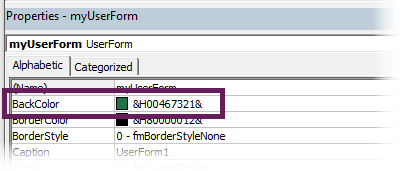The color code in the screenshot above is: &H00467321&.  However, there is a very subtle difference when using the VBA code… the hex code needs to be reversed.  The first two characters represent blue, the middle two characters represent green and last two are blue.

Normally, blue would be #0000FF, but for the VBA property the code would be: &H00FF0000&

• & = Start character
• H = Hex code
• 00 = Tells VBA to use a custom color
• FF0000 = the Hex color code with the RGB reversed
• & = End character

### CMYK

Another common color code system is CMYK.  It is used primarily for printing, as it determines the amount of Cyan, Magenta, Yellow and blacK ink used in overlapping printed dots.

While Excel does not use CMYK, it is a common method, so I have included it within the scope of this post.## User Defined Functions for color conversion

In the section above, I’ve tried to explain how each method defines colors, but converting between them is a different matter entirely, and is much harder.  Thankfully, we can let Excel do the work for us.  Below are the UDF’s to convert between the various methods.

REMEMBER! – To use VBA functions, the code must be included in a standard VBA code module.

RGB, hex and long are all based on whole numbers, so they should perfectly convert between each other.  CMYK and HSL involve decimal places and percentages and therefore could create small differences in their conversions.

### Convert from RGB to hex

The following UDF calculates the hex value based on the RGB color codes.

```Function GetHexFromRGB(Red As Integer, Green As Integer, Blue As Integer) As String

GetHexFromRGB = "#" & VBA.Right\$("00" & VBA.Hex(Red), 2) & _
VBA.Right\$("00" & VBA.Hex(Green), 2) & VBA.Right\$("00" & VBA.Hex(Blue), 2)

End Function```

How to use the function:

The function contains 3 arguments, values between 0 and 255 for each individual Red, Green, or Blue value.### Convert from hex to RGB

The UDF below provides the Red, Green, or Blue value based on the hex color.

```Function GetRGBFromHex(hexColor As String, RGB As String) As String

hexColor = VBA.Replace(hexColor, "#", "")
hexColor = VBA.Right\$("000000" & hexColor, 6)

Select Case RGB

Case "B"
GetRGBFromHex = VBA.Val("&H" & VBA.Mid(hexColor, 5, 2))

Case "G"
GetRGBFromHex = VBA.Val("&H" & VBA.Mid(hexColor, 3, 2))

Case "R"
GetRGBFromHex = VBA.Val("&H" & VBA.Mid(hexColor, 1, 2))

End Select

End Function```

How to use the function:

The function contains 2 arguments;

1. the hex code
2. the letter R, G or B, to represent the color to be returned### Convert from RGB to long

This UDF provides the long value based on the RGB color codes.

```Function GetLongFromRGB(Red As Integer, Green As Integer, Blue As Integer) As Long

GetLongFromRGB = RGB(Red, Green, Blue)

End Function```

How to use the function:

The function contains 3 arguments, values between 0 and 255 for each individual Red, Green, or Blue value.### Convert from long to RGB

To convert from RGB to the long color code, use the following UDF.

```Function GetRGBFromLong(longColor As Long, RGB As String) As Integer

Select Case RGB

Case "R"
GetRGBFromLong = (longColor Mod 256)

Case "G"
GetRGBFromLong = (longColor \ 256) Mod 256

Case "B"
GetRGBFromLong = (longColor \ 65536) Mod 256

End Select

End Function```

How to use the function:

The function contains 2 arguments;

1. the long code
2. the letter R, G, or B, depending on which color we want to return.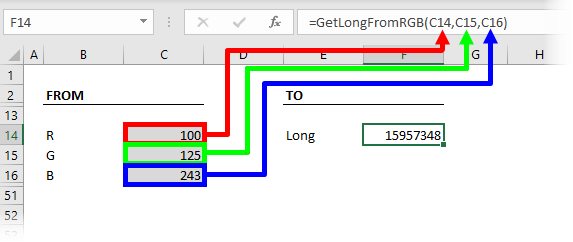### Convert from long to hex

The following UDF provides the hex code based on the long color code.

```Function GetHexFromLong(longColor As Long) As String

Dim R As String
Dim G As String
Dim B As String

R = Format(Application.WorksheetFunction.Dec2Hex(longColor Mod 256), "00")
G = Format(Application.WorksheetFunction.Dec2Hex((longColor \ 256) Mod 256), "00")
B = Format(Application.WorksheetFunction.Dec2Hex((longColor \ 65536) Mod 256), "00")

GetHexFromLong = "#" & R & G & B

End Function```

How to use the function:

The function has only one argument, which is the long color code.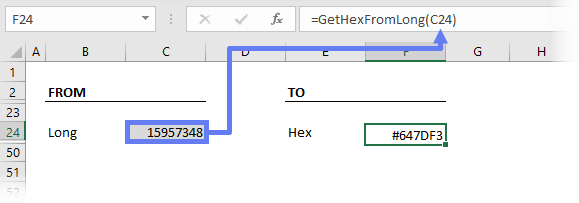### Convert from hex to long

The UDF below converts the hex code into the long color code.

```Function GetLongFromHex(hexColor As String) As Long

Dim R As String
Dim G As String
Dim B As String

hexColor = VBA.Replace(hexColor, "#", "")
hexColor = VBA.Right\$("000000" & hexColor, 6)

R = Left(hexColor, 2)
G = Mid(hexColor, 3, 2)
B = Right(hexColor, 2)

GetLongFromHex = Application.WorksheetFunction.Hex2Dec(B & G & R)
End Function```

How to use the function:

The function has only one argument, which is the hex code.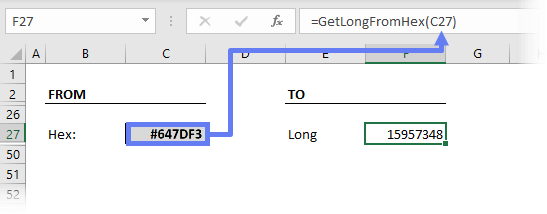### Convert from RGB to HSL

The UDF below will return the degree of Hue, % of Saturation, or % of Luminance based on the RGB color codes.

```Function GetHSLFromRGB(Red As Integer, Green As Integer, Blue As Integer, _
HSL As String)

Dim RedPct As Double
Dim GreenPct As Double
Dim BluePct As Double
Dim MinRGB As Double
Dim MaxRGB As Double
Dim H As Double
Dim S As Double
Dim L As Double

RedPct = Red / 255
GreenPct = Green / 255
BluePct = Blue / 255

MinRGB = Application.WorksheetFunction.Min(RedPct, GreenPct, BluePct)
MaxRGB = Application.WorksheetFunction.Max(RedPct, GreenPct, BluePct)

L = (MinRGB + MaxRGB) / 2

If MinRGB = MaxRGB Then
S = 0
ElseIf L < 0.5 Then
S = (MaxRGB - MinRGB) / (MaxRGB + MinRGB)
Else
S = (MaxRGB - MinRGB) / (2 - MaxRGB - MinRGB)
End If

If S = 0 Then
H = 0
ElseIf RedPct >= Application.WorksheetFunction.Max(GreenPct, BluePct) Then
H = (GreenPct - BluePct) / (MaxRGB - MinRGB)
ElseIf GreenPct >= Application.WorksheetFunction.Max(RedPct, BluePct) Then
H = 2 + (BluePct - RedPct) / (MaxRGB - MinRGB)
Else
H = 4 + (RedPct - GreenPct) / (MaxRGB - MinRGB)
End If

H = H * 60

If H < 0 Then H = H + 360

Select Case HSL

Case "H"
GetHSLFromRGB = H

Case "S"
GetHSLFromRGB = S

Case "L"
GetHSLFromRGB = L

End Select

End Function```

How to use the function:

The function contains 4 arguments.  The R, G and B values, then the letter H, S, or L, depending on the value to be returned by the function.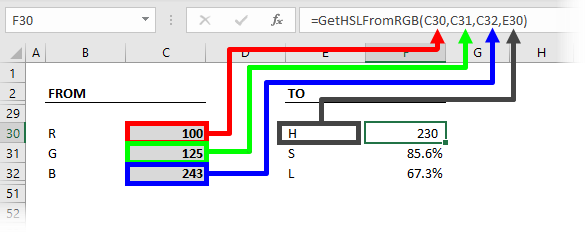The calculation for converting to HSL is based on the calculation from here: http://www.niwa.nu/2013/05/math-behind-colorspace-conversions-rgb-hsl/

### Convert from HSL to RGB

The following UDF calculates the RGB values based on the degree of Hue, a% of Saturation % of Luminance

```Function GetRGBFromHSL(Hue As Double, Saturation As Double, Luminance As Double, _
RGB As String)

Dim R As Double
Dim G As Double
Dim B As Double
Dim temp1 As Double
Dim temp2 As Double
Dim tempR As Double
Dim tempG As Double
Dim tempB As Double

If Saturation = 0 Then

R = Luminance * 255
G = Luminance * 255
B = Luminance * 255

GoTo ReturnValue

End If

If Luminance < 0.5 Then
temp1 = Luminance * (1 + Saturation)
Else
temp1 = Luminance + Saturation - Luminance * Saturation
End If

temp2 = 2 * Luminance - temp1

Hue = Hue / 360

tempR = Hue + 0.333
tempG = Hue
tempB = Hue - 0.333

If tempR < 0 Then tempR = tempR + 1
If tempR > 1 Then tempR = tempR - 1
If tempG < 0 Then tempG = tempG + 1
If tempG > 1 Then tempG = tempG - 1
If tempB < 0 Then tempB = tempB + 1
If tempB > 1 Then tempB = tempB - 1

If 6 * tempR < 1 Then
R = temp2 + (temp1 - temp2) * 6 * tempR
Else
If 2 * tempR < 1 Then
R = temp1
Else
If 3 * tempR < 2 Then
R = temp2 + (temp1 - temp2) * (0.666 - tempR) * 6
Else
R = temp2
End If
End If
End If

If 6 * tempG < 1 Then
G = temp2 + (temp1 - temp2) * 6 * tempG
Else
If 2 * tempG < 1 Then
G = temp1
Else
If 3 * tempG < 2 Then
G = temp2 + (temp1 - temp2) * (0.666 - tempG) * 6
Else
G = temp2
End If
End If
End If

If 6 * tempB < 1 Then
B = temp2 + (temp1 - temp2) * 6 * tempB
Else
If 2 * tempB < 1 Then
B = temp1
Else
If 3 * tempB < 2 Then
B = temp2 + (temp1 - temp2) * (0.666 - tempB) * 6
Else
B = temp2
End If
End If
End If

R = R * 255
G = G * 255
B = B * 255

ReturnValue:
Select Case RGB

Case "R"
GetRGBFromHSL = Round(R, 0)

Case "G"
GetRGBFromHSL = Round(G, 0)

Case "B"
GetRGBFromHSL = Round(B, 0)

End Select

End Function```

How to use the function:

The function contains 4 arguments.  The Hue degree, Saturation %, and Luminance %, plus the letter R, G or B depending on the value to be returnedThe calculation for converting from HSL is based on the calculation from here: http://www.niwa.nu/2013/05/math-behind-colorspace-conversions-rgb-hsl/

### Convert from RGB to CMYK

The following UDF provides the % value for Cyan, Magenta, Yellow, or Black based on the RGB color codes.

```Function GetCMYKFromRGB(Red As Integer, Green As Integer, Blue As Integer, _
CMYK As String) As Double

Dim K As Double
Dim RedPct As Double
Dim GreenPct As Double
Dim BluePct As Double
Dim MaxRGB As Double

RedPct = Red / 255
GreenPct = Green / 255
BluePct = Blue / 255
MaxRGB = Application.WorksheetFunction.Max(RedPct, GreenPct, BluePct)

If MaxRGB = 0 And CMYK <> "K" Then
GetCMYKFromRGB = 0
Exit Function
End If

K = 1 - MaxRGB

Select Case CMYK

Case "C"
GetCMYKFromRGB = (1 - RedPct - K) / (1 - K)

Case "M"
GetCMYKFromRGB = (1 - GreenPct - K) / (1 - K)

Case "Y"
GetCMYKFromRGB = (1 - BluePct - K) / (1 - K)

Case "K"
GetCMYKFromRGB = K

End Select

End Function```

How to use the function:

The function requires 4 arguments.  The R, G and B values, along with the letter C, M, Y or K, depending on the value to be returned.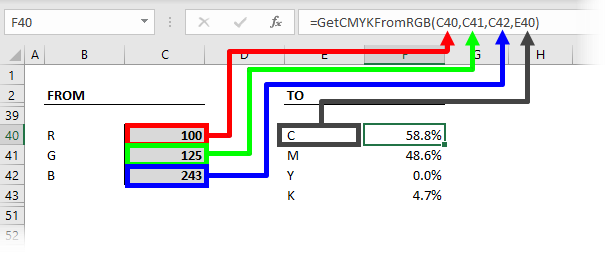The calculation for converting to CMYK  is based on the calculation from here: https://www.easycalculation.com/colorconverter/cmyk-rgb-color-convertor.php

### Convert from CMYK to RGB

To get the C, M, Y, or K percentages from RGB use the UDF below.

```Function GetRGBFromCMYK(C As Double, M As Double, Y As Double, K As Double, _
RGB As String) As Integer

Select Case RGB

Case "R"
GetRGBFromCMYK = 255 * (1 - K) * (1 - C)

Case "G"
GetRGBFromCMYK = 255 * (1 - K) * (1 - M)

Case "B"
GetRGBFromCMYK = 255 * (1 - K) * (1 - Y)

End Select

End Function```

How to use the function:

The function requires 5 arguments.  The Cyan, Magenta, Yellow and Black percentages, plus the letter R, G or B, depending on the value to be returned.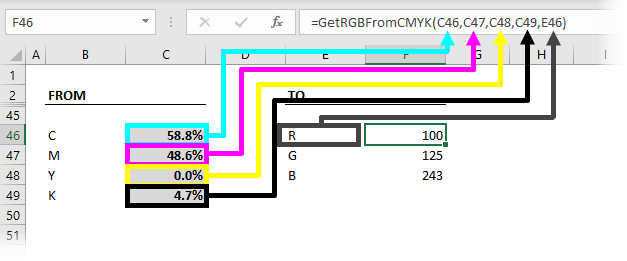The calculation for converting from CMYK  is based on the calculation from here: https://stackoverflow.com/questions/10690125/coloring-cells-in-excel-with-cmyk-cell-values

## Color conversion add-in

Using these conversion functions, I have created an Excel add-in that will quickly switch between the different color codes.

To install the add-in, follow the PDF instructions included in the download, or follow the instructions in this post.

Once installed, the EOTG menu will include an icon called Converter in the Color Codes group.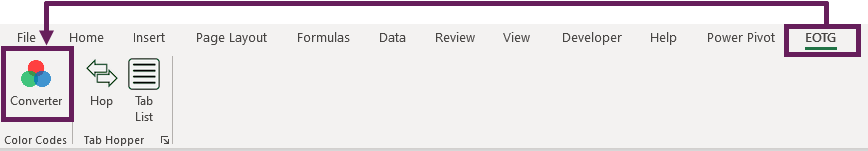Click the button to open the tool.  When entering values into any of the boxes, the other boxes will automatically display the converted codes.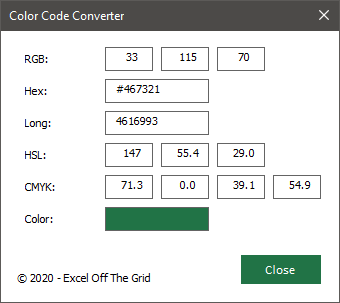## Conclusion

I hope this post provides you with all the color conversions that you need.  If you want to convert between two methods that I have not covered above (for example, hex to HSL has not been included), convert from the source code to RGB, then from RGB to the target mode.Get our FREE VBA eBook of the 30 most useful Excel VBA macros.

Automate Excel so that you can save time and stop doing the jobs a trained monkey could do.

Don’t forget:

If you’ve found this post useful, or if you have a better approach, then please leave a comment below.

Do you need help adapting this to your needs?

I’m guessing the examples in this post didn’t exactly meet your situation.  We all use Excel differently, so it’s impossible to write a post that will meet everybody’s needs.  By taking the time to understand the techniques and principles in this post (and elsewhere on this site) you should be able to adapt it to your needs.

But, if you’re still struggling you should:

1. Read other blogs, or watch YouTube videos on the same topic.  You will benefit much more by discovering your own solutions.
2. Ask the ‘Excel Ninja’ in your office.  It’s amazing what things other people know.
3. Ask a question in a forum like Mr Excel, or the Microsoft Answers Community.  Remember, the people on these forums are generally giving their time for free.  So take care to craft your question, make sure it’s clear and concise.  List all the things you’ve tried, and provide screenshots, code segments and example workbooks.
4. Use Excel Rescue, who are my consultancy partner.   They help by providing solutions to smaller Excel problems.

What next?
Don’t go yet, there is plenty more to learn on Excel Off The Grid.  Check out the latest posts:

## 12 thoughts on “Convert color codes (RGB, HSL, Hex, Long, CMYK)”

1.Anon says:

GetHSLFromRGB doesn’t always show correct hue e.g. RGB(128,128,0) should convert to 60 degrees but it returns 240 degrees.

•Excel Off The Grid says:

Ah… yes, you’re right. Thank you for letting me know.

I managed to miss out an equals symbol in the calculation. Hopefully, it should be calculating correctly now.

2.Michael D Crowe says:

Hex codes are not in the reverse order of RGB! #FF0000 is absolutely red and #0000FF is blue.

•Excel Off The Grid says:

Eeek – how did that sneak through. Thanks for letting me know. No fixed 🙂

3.Anonymous says:

Thank you for this add-in. I tried to make some VBA modifications to the add-in but a “Project is unviewable” message comes up. Is it possible to modify it?

•Excel Off The Grid says:

The file has been protected. All the calculations used are contained within the article.

4.Jorge Cabral says:

Hi

It’s not possible to get the file, unprotected, to make some changes?

Thanks

•Excel Off The Grid says:

I have protected the file to prevent changes. All the codes used for the calculations are included in the article, other interface should be easy enough to replicate with a few mins of work.

5.Nico says:

Hi,
Does it work with Google sheet ?

•Excel Off The Grid says:

I’m sure it is possible to amend the code examples to work with Google Sheets. But I don’t know what changes you would need to make, it’s been a long while since I’ve used Google Sheets.

6.Nico says:

Hi,

Interested in RGB to CMYK convertion, is there a way to adapt it for googlesheet please?
The macro doesn’t seem to work in GoogleSheet

•Excel Off The Grid says:

The calculations are visible in the example code for you to see. You should be able to use that as a base to create something similar for Google Sheets.